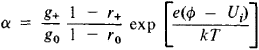# Langmuir-Saha Equation

The following article is from The Great Soviet Encyclopedia (1979). It might be outdated or ideologically biased.

## Langmuir-Saha Equation

an equation that defines the degree of ionization a of the vapors of a substance by the heated surface of a metalin contact with the vapors. The equation was derived by I. Langmuir in 1924, using results previously obtained by M. Saha. The particles of the vapor at first “adhere” to the surface of the metal and then vaporize from it. If n and n+ are the quantities of neutral atoms and positive ions of the substance that evaporate in 1 sec per unit area, then α = n+//n. The Langmuir-Saha equation has the formwhere e is the electron charge, Ui is the ionization potential of the given substance, r0 and r+ are the coefficients of reflection of its atoms and positive ions from the surface of the metal, T is the absolute temperature of the surface, Φ is the work function of the metal, k is the Boltzmann constant, and g+ and g0 designate, respectively, the number of different states of positive ions and neutral atoms of the substance that have identical energies corresponding to the temperature T. The Langmuir-Saha equation describes positive surface ionization; a similar equation, sometimes also called the Langmuir-Saha equation, is valid for the process in which negative ions are formed.